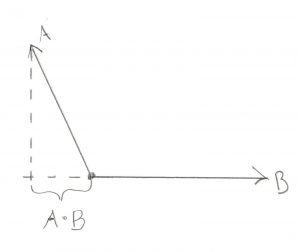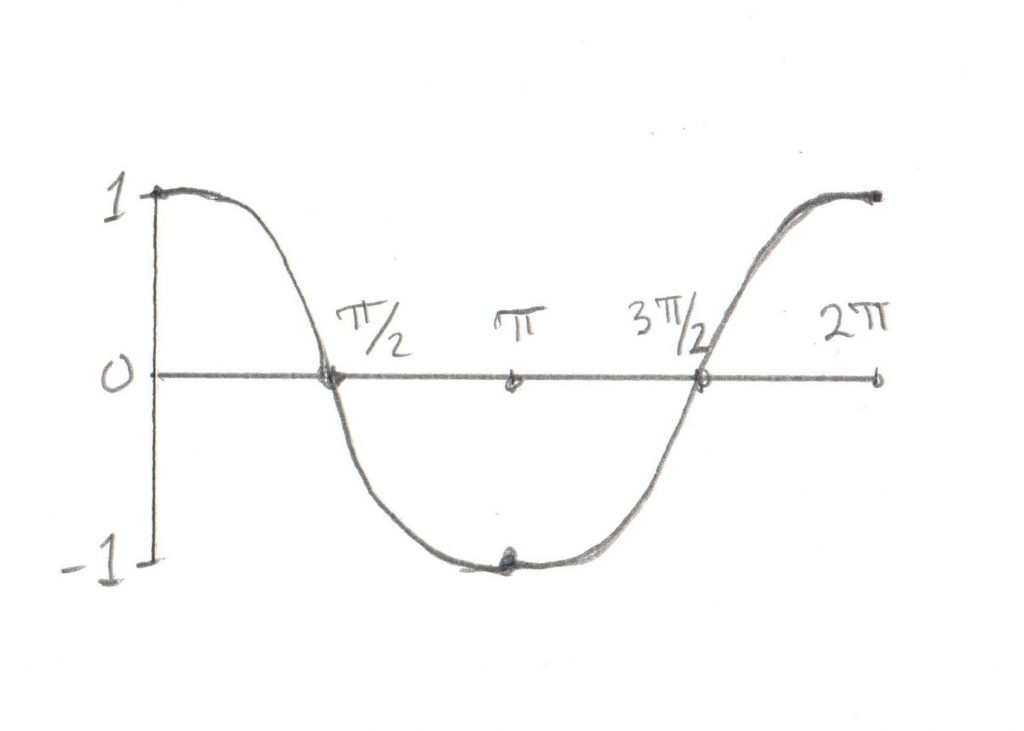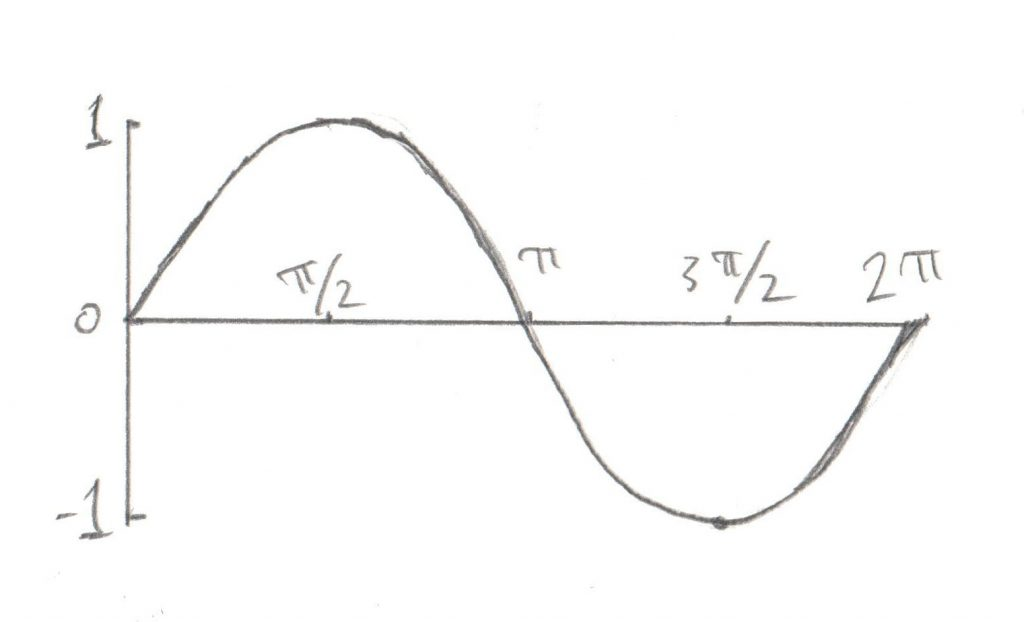# Vector Math

Okay, for this post let’s finally stop talking about the Philosophy behind the class design, and instead look at some math. We’re going to start with the basics of Vector Math and build up a working vocabulary of what we can do with it.

A Vector is a direction and a magnitude, represented by 3 floating points. This could mean something as simple as:

```struct Vector {
Vector( float x_coord, float y_coord, float z_coord )
: x( x_coord )
, y( y_coord )
, z( z_coord )
{}
float x;
float y;
float z;
};
```

I’m going to assume that you’re comfortable with the idea of 3D and x, y, and z axis. What do I mean when I say a Vector is a direction AND a magnitude? Well consider these 3 Vectors

```    Vector v1( 1, 0, 0 );
Vector v2( 2, 0, 0 );
Vector v3( 0, 1, 0 );
```

`v1` and `v2` have the same direction, which is directly along the x axis. However `v1` is 1 unit long, while `v2` is 2 units long. `v3` has the same length as `v1` (1 unit) but its direction is different, it’s along the y axis.

You’ll remember from my post about Points and Vectors that while Points also have 3 floats, their meaning is different. A Point does not represent a direction and magnitude, it represents a position in space.

Now then, the simplest set of operations on Vectors are addition and subtraction

```Vector operator+(const Vector & a, const Vector & b)
{
return Vector(a.x + b.x, a.y + b.y, a.z + b.z);
}

Vector operator-(const Vector & a, const Vector & b)
{
return Vector(a.x - b.x, a.y - b.y, a.z - b.z);
}
```

Vectors can also be multiplied and divided by a scalar (a single float)

```Vector operator*( const Vector& a, float b )
{
return Vector( a.x*b, a.y*b, a.z*b );
}

Vector operator/( const Vector& a, float b )
{
return Vector( a.x/b, a.y/b, a.z/b );
}
```

Not much magic here, this shouldn’t be very surprising.

The next bit to consider is a Vector’s length. This can be found using this pair of functions

```float Vector::length_squared() const
{
return x*x + y*y + z*z;
}

float Vector::length() const
{
return sqrt(length_squared());
}
```

If you’re unfamiliar with this code you may be wondering why there’s a `float length_squared()` function. The reason is an optimization. Now I’m definitely not one to optimize prematurely, and I have never actually profiled how much more time it takes to perform the extra square root functions, however there are times when you really don’t care how long the actual length is, you just need to compare it to another Vector’s length. For example, given three Vectors A, B, and C is the length from A to B longer then the length from A to C? We don’t care what the length is, we just need to know which is longer. Comparing the `length_squared` will get us the same result.

Okay, now let’s move on to something that you might not remember so well from High School Math, the dot product and cross product.

```float dot_product( const Vector& a, const Vector& b )
{
return a.x*b.x + a.y*b.y + a.z*b.z;
}

Vector cross_product( const Vector& a, const Vector& b )
{
return Vector( (a.y*b.z) - (a.z*b.y),
(a.z*b.x) - (a.x*b.z),
(a.x*b.y) - (a.y*b.x));
}
```

So the math isn’t so daunting, but what do these things mean? Well that starts to get interesting.

# Dot Product

The dot product of 2 Vectors is (sorta) the length of the projection of the first vector on the second one. For now let’s not dwell on the actual value of the length, but let’s get an intuitive feel by looking at two examples:

In the first image you can see that the two Vectors mostly overlap each other, so the dot product is “big” (again, not worrying about the actual numerical value). In the second image, the two Vectors don’t overlap much, so the dot product is “little.” By checking to see if the dot product is “big” or “little” you can start to get an intuition of how much the Vectors overlap.

What if the Vectors don’t overlap?In this case the dot product will be negative. How is this useful? Turns out it’s really useful. Let’s say you’re writing a space ship video game (I love space ship video games) and you have one Vector pointing directly forward from your ship. Then you have another Vector pointing towards an attacker. Is the attacker in front of you or behind you? Seems like a pretty important thing to know. Well if the dot product of the two Vectors is positive then the attacker is in front of you. If it’s negative then the attacker is behind.

That’s not a bad start, but what about the actual length itself? I’ve been saying the dot product is “sorta” the length, well what’s the actual length? It turns out to be related to the cosine of the angle between the two Vectors

```// not actually valid C++
cos(angle) = dot_product(a,b) / (a.length() * b.length())
```

Okay, let’s try to give you a gut feel for how this makes sense. Do you remember your cosine curve from trigonometry class? If not let me highlight the important bits:angle (degrees) cosine 0 1 90 (radians: pi/2) 0 180 (radians: pi) -1 270 (radians: 3pi/2) 0

Now think about our two Vectors. If the angle between them is 0 then they completely overlap, so the projection of one Vector on the other is exactly the length of the Vector itself (so when you divide it by the Vector length you get 1, which corresponds to our cosine table). Now how about if we look at the dot product of two Vectors at 90 degrees to one another. There is no overlap at all, the length of the projection is 0, which is exactly the value of the cosine of 90 degrees from our table. If the two Vectors point in exactly the opposite way from one another, then the overlap is negative 1, etc etc. I don’t want to kick this dead horse, but getting an intuitive feel for this will be very helpful. If you feel like your head is spinning a bit, get yourself a pencil and paper and re-read this section while drawing out your own examples. Trust me, it’s worth the time to have a feel for this.

# Cross Product

So with the dot product put to rest it’s time to tackle the big one, the cross product. Unfortunately this one is a bit harder to picture because the cross product of two Vectors is yet another Vector. To start to visualize this, first get comfortable with the fact that any two Vectors describe a plane. It doesn’t matter how you draw them, two Vectors with the same origin will always be in the same plane. The cross product of any two Vectors is a third Vector that is perpendicular to that plane. Again, let’s not worry about how long that Vector is, it’s important to know that it’s perpendicular to the other two. The length itself is proportional to the angle between those two Vectors:

Notice that in the first drawing the two Vectors overlap a lot (ie their dot product is “big”) and the third Vector, the cross product, is not very long, it’s “little.” Conversely in the second drawing the two Vectors don’t overlap much at all (dot product is “little”) and the third vector is long, or “big.”

So how is this useful? Well to understand that we need to understand Rotation Vectors, which I will be covering in a later section. I don’t want to confuse this section too much, so for now just trust me that the cross product is helpful. After you’ve read about Rotation Vectors, come back here and see if you can figure it out.

How about we try to put a concrete number on how “big” or “little” our cross products are? Well in this case the math is:

```// not actually valid C++
sin(angle) = cross_product( a, b ).length() / (a.length() * b.length())
```

Okay, so in this case the length of the Vector created by the cross product is related to the sine of the angle between the Vectors. How much do you remember your sine curve? Here’s the important bits:angle (degrees) sine 0 0 90 (radians: pi/2) 1 180 (radians: pi) 0 270 (radians: 3pi/2) -1

In this case when the two Vectors completely overlap, the length of the third Vector (the cross product) is 0. As the angle between them grows to 90 degrees, the length of the third Vector grows to the maximum. As the angle goes past 90 degrees, the length of the third Vector (the cross product) goes back to 0. Again, if you have trouble imagining this in your head, get a pencil and paper and try drawing out a few examples. As with the dot product, it’s important to have a good gut feeling for this

Okay, that was a lot of math, but hopefully worth it. Once it starts to make sense, have a look at my code to see how it can be written. Again, for now ignore that my Vectors and Unit_vectors are templates, we’ll discuss why that is in a later post:

• Triple – the basics, addition and subtraction
• Vector – multiplication with scalars and lengths
• Unit_vector – most operations result in non-unit Vectors
• Vector_math – dot product and cross product
 << Vector Types Contents Rotations >>